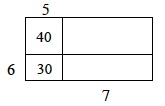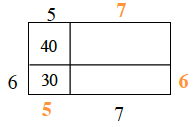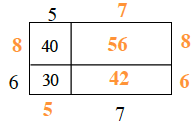### Home > AC > Chapter 2 > Lesson 2.1.1 > Problem2-9

2-9.

Find the perimeter of the entire rectangle shown below (that is, the outside boundary of the figure). Notice that the areas of two of the parts have been labeled inside the rectangle. Also find the total area. Remember to show all work leading to your solution.Remember that the numbers within the boxes are the products of its dimensions. Using that information, attempt to find the remaining dimensions of the rectangle.

Because the shape is a rectangle by definition, the opposite sides are equal in length.

Using the information given above, label the parts of the rectangle that can be found just by looking at the shape.

Now find the pieces not given.
$5(?)=40$
$6\cdot7=42$
$8\cdot7=56$Find the perimeter and area using the strategy shown in problem 2-8.

perimeter $=52$ units
area $=168$ units2